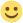# Posts by Oli E Lee

Got it, I hope!

So we're looking for the time that the distance is 50km. Your army travels at 25 km/r. Enemy army travels at 35% of 25km/r (25*0.35) which is 8.75 km/h. Attack range is 50km. This means that you're 50km + 25km, so 75km away to start with.

Looking for the hours it takes for the armies to get exactly 50km apart...

x is the number of hours.

(75 + 8.75x) - 25x = 50

75 + 8.75x = 50 + 25x

25 = 16.25x

x = 1.538461538461538...

Convert that to HH:MM:SS = 1hr 32m 18s

This was a fun puzzle, but maybe wrongYour army travels at 70% of 25km/r (25*0.7). Enemy army travels at 35% of 25km/r (25*0.35). Attack range is 50km. This means that you're 50km + 17.5km (one hour march of 25*0.7), so 67.5km to start with.

Looking for the hours it takes for the armies to catch...

x is the number of hours.

x(25*0.7) = 67.5 + (x(25*0.35)).

17.5x = 67.5 + 8.75x

8.75x = 67.5

x = 7.71428571428571

Covert that to HH:MM:SS = 7hrs 42mins 52 seconds